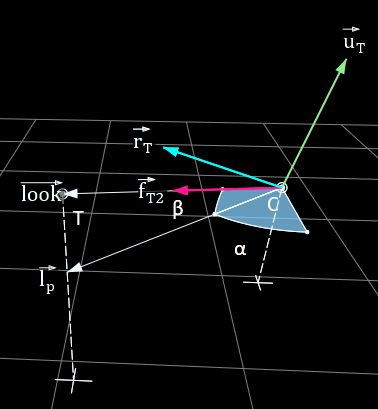/
DISCIPLINES

# Quaternion rotations over two axes

In this blog post I derive a quaternion formula for a class of rotations which involves only two axes that can be applied directly to rotate a camera or turret system.

# Introduction

In my teaching experience, most students learn to work with transformation hierarchies and how to work with them to achieve a desired transformation result. However, there is one class of problems which causes a lot of delays and frustration, namely a rotation over two axes where the second axis is dependent on the rotation of the first axis.

To be very clear, this is not another way to define Euler angles, since an euler is a hierarchy of rotations around independent axes. In other words if an Euler rotation is defines as first over X, then over Y and Z last, then the X-rotation has no influence over the axis of rotation of Y.

For example, a camera rotation can be defined as a rotation over the up-axis of the world coordinate system, and by a second rotation over the rotated right vector, as shown in the following image where $$\alpha$$ is the rotation over the world up axis and and $$\beta$$ is the rotation over the rotated right axis $$\vec{r_T}$$:The order of these two rotations ensures that the right vector $$\vec{r_T}$$ remains parallel with the world ground plane, in other words the operation does not introduce a tilt in the camera system.

Other examples are rotations of a tank turret and rotations of a joint system with two degrees of freedom.

# Mathematical derivation

The quaternion formula is relatively simple to derive and leads to a simple and elegant quaternion formula. First we define two quaternions that describe the rotations:

$$q_1 = [ 0,0,sin(\frac{\alpha}{2}),cos(\frac{\alpha}{2})] \\ q_2 = [cos(\alpha)sin(\frac{\beta}{2}),sin(\alpha)sin(\frac{\beta}{2}) ,0,cos(\frac{\beta}{2})]$$

The axis of rotation of the first quaternion $$q_1$$is (0,0,1) and the angle to rotate over this axis is $$\alpha$$. For the second rotation we rotate over the rotated X-axis:

$$\vec{r_{T}} = q_1 [1,0,0,0] q_1^* = [cos(\alpha),sin(\alpha),0,0]$$

Using this rotation axis $$\vec{r_T}$$ over an angle of $$\beta$$ results in the second quaternion $$q_2$$.

The combined rotation is then:

$$q_T = q_2 * q_1$$

Working out this product results in the following quaternion:

$$q_T = \begin{pmatrix} cos (\frac{\alpha}{2})cos(\alpha)sin(\frac{\beta}{2})+ sin (\frac{\alpha}{2})sin(\alpha)sin(\frac{\beta}{2}) \\ cos (\frac{\alpha}{2})sin(\alpha)sin(\frac{\beta}{2})- sin (\frac{\alpha}{2})cos(\alpha)sin(\frac{\beta}{2})\\ sin(\frac{\alpha}{2})cos(\frac{\beta}{2})\\ cos(\frac{\alpha}{2})cos(\frac{\beta}{2}) \end{pmatrix}$$

In the i and j components we can isolate $$sin(\frac{\beta}{2})$$ and apply Simpson product formulas:

$$q_T = \begin{pmatrix} sin(\frac{\beta}{2})( \frac{cos (\frac{\alpha}{2})+cos (\frac{3\alpha}{2})+cos (\frac{\alpha}{2})-cos (\frac{3\alpha}{2}) }{2}) \\ sin(\frac{\beta}{2})( \frac{sin (\frac{\alpha}{2})+sin (\frac{3\alpha}{2})+sin (\frac{\alpha}{2})-sin (\frac{3\alpha}{2}) }{2})\\ sin(\frac{\alpha}{2})cos(\frac{\beta}{2}) \\ cos(\frac{\alpha}{2})cos(\frac{\beta}{2}) \end{pmatrix}$$

Which leads to the final formula:

$$q_T = \begin{pmatrix} cos (\frac{\alpha}{2}) sin(\frac{\beta}{2}) \\ sin (\frac{\alpha}{2}) sin(\frac{\beta}{2})\\ sin(\frac{\alpha}{2})cos(\frac{\beta}{2}) \\ cos(\frac{\alpha}{2})cos(\frac{\beta}{2}) \end{pmatrix}$$

I hope the reader agrees that this is an interesting and elegant formula to use.

## Numerical examples

A couple of numerical examples are given to validate the formula and provide a way to test possible implementations of this formula:

Numerical examples
$$\alpha$$ $$\beta$$ $$q_2$$ $$q_1$$ $$q_2 * q_1$$ $$q_T$$
$$\pi/6$$ $$\pi/3$$ $$\begin{pmatrix} 0.433 \\ 0.250 \\ 0.000 \\ 0.866 \end{pmatrix}$$ $$\begin{pmatrix} 0.000 \\ 0.000 \\ 0.259 \\ 0.966 \end{pmatrix}$$ $$\begin{pmatrix} 0.483 \\ 0.129 \\ 0.224 \\ 0.837 \end{pmatrix}$$ $$\begin{pmatrix} 0.483 \\ 0.129 \\ 0.224 \\ 0.837 \end{pmatrix}$$
$$\pi/4$$ $$\pi/6$$ $$\begin{pmatrix} 0.183 \\ 0.183 \\ 0.000 \\ 0.966 \end{pmatrix}$$ $$\begin{pmatrix} 0.000 \\ 0.000 \\ 0.383 \\ 0.924 \end{pmatrix}$$ $$\begin{pmatrix} 0.239 \\ 0.099 \\ 0.370 \\ 0.892 \end{pmatrix}$$ $$\begin{pmatrix} 0.239 \\ 0.099 \\ 0.370 \\ 0.892 \end{pmatrix}$$
$$-\pi/8$$ $$5\pi/6$$ $$\begin{pmatrix} 0.892 \\ -0.370 \\ 0.000 \\ 0.259 \end{pmatrix}$$ $$\begin{pmatrix} 0.000 \\ 0.000 \\ -0.195 \\ 0.981 \end{pmatrix}$$ $$\begin{pmatrix} 0.947 \\ -0.188 \\ -0.050 \\ 0.254 \end{pmatrix}$$ $$\begin{pmatrix} 0.947 \\ -0.188 \\ -0.050 \\ 0.254 \end{pmatrix}$$

# Quaternion analysis

It is also possible to check wether or not a quaternion was defined with the formula as derived. We start from the formula itself:

$$q_T = \begin{pmatrix} cos (\frac{\alpha}{2}) sin(\frac{\beta}{2}) \\ sin (\frac{\alpha}{2}) sin(\frac{\beta}{2})\\ sin(\frac{\alpha}{2})cos(\frac{\beta}{2}) \\ cos(\frac{\alpha}{2})cos(\frac{\beta}{2}) \end{pmatrix}$$

First we take a look of the ratios of the y and x component and the z and w component:

$$\dfrac{q_y}{q_x} = \dfrac{sin(\alpha/2)}{cos(\alpha/2)} = tan(\alpha/2) \\ \dfrac{q_z}{q_w} = \dfrac{sin(\alpha/2)}{cos(\alpha/2)} = tan(\alpha/2) \\$$

It is clear that the ratios have to be the same if the quaternion defines a rotation as calculated by $$q_T$$

With the same reasoning we can calculate the ratios of the x and w component and the y and z components, and this is a second condition that needs to hold if the rotation is a rotation over two axes:

$$\dfrac{q_x}{q_w} = \dfrac{sin(\beta/2)}{cos(\beta/2)} = tan(\beta/2) \\ \dfrac{q_y}{q_z} = \dfrac{sin(\beta/2)}{cos(\beta/2)} = tan(\beta/2) \\$$

So for a rotation that is defined as a rotation over the Z-axis, followed by a rotation over the rotated X-axis we can conclude that it is possible to determine if a random quaternion satisfies the formula, and furthermore, we can easily calculate the angles $$\alpha$$ and $$\beta$$ that were used to construct this quaternion by using the atan2 function:

$$\alpha = atan2(q_y, q_x) = atan2(q_z,q_w) \\ \beta = atan2(q_x,q_w)= atan2(q_y,q_z)$$

# References

Simpson formula:
https://proofwiki.org/wiki/Simpson%27s_Formulas
Quaternion formula:
https://www.gamasutra.com/view/feature/131686/rotating_objects_using_quaternions.php

### Latest Jobs

#### Sucker Punch Productions

Bellevue, Washington
08.27.21
Combat Designer

#### Xbox Graphics

Redmond, Washington
08.27.21
Senior Software Engineer: GPU Compilers

#### Insomniac Games

Burbank, California
08.27.21
Systems Designer

#### Deep Silver Volition

Champaign, Illinois
08.27.21
Senior Environment Artist
More Jobs